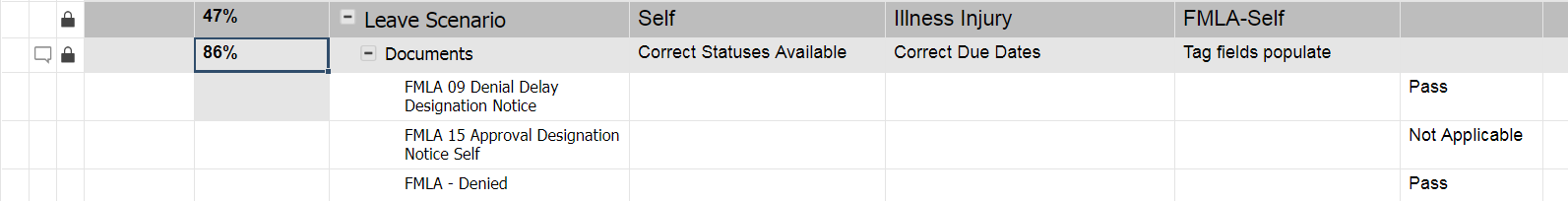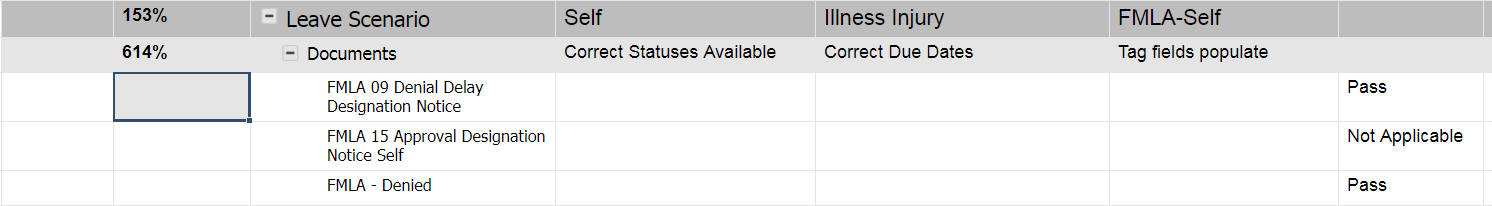or Explore Discussions

# Formula using Sum and Countif not returning expected outcomes

03/28/18 Edited 12/09/19

Here's a version of the formula that works. However I need to include a second value and get an error when I try. I'm sure it's the formula syntax. Not sure if there is a better way to get the right outcome. I basically am trying to determine % Complete OR Not Applicable

Working with just Pass as a value

=SUM((COUNTIF(CHILDREN(Status22), ="Pass")) / SUM(COUNT(CHILDREN(Status22))))

When a Not Applicable is selected the above formula results in a reduction to the % Complete and the desired outcome would be for Pass and Not Applicable to both count so if a pass or not applicable are used the % complete would be the same.

=SUM((COUNTIF(CHILDREN(Status22), ="Pass") + (COUNTIF(CHILDREN(Status22), ="Not Applicable")) / SUM(COUNT(CHILDREN(Status22))))

When I use the formula above I get a number much higher than 100% which is the max I should be getting

Not sure the right way to structure the formula to get a % complete based on the sum of both Pass and Not Applicable versus overall number of children. Which is how I think the formula should be structured. Any suggestions?Popular Tags:

• Without taking a hard look at it... try this revision?

=COUNTIF(CHILDREN(Status22), ="Pass") + COUNTIF(CHILDREN(Status22), ="Not Applicable") / COUNT(CHILDREN(Status22))

You don't need all the SUM's cause the countif will automatically sum it for you and you're doing simple math to add and then divide each part.

• Th formula you gave me did not work. It returned 700%.

However the following formula did work

=SUM(COUNTIF(CHILDREN(Status22), ="Pass") + COUNTIF(CHILDREN(Status22), ="Not Applicable")) / COUNT(CHILDREN(Status22))

Thank you!

• Hmmm. Cool. Glad I helped you get on the right track!You're welcome!